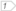#Boost C++ Libraries

...one of the most highly regarded and expertly designed C++ library projects in the world.

### Unconstrained Sets

Unconstrained sets allow the user to disable one of the views of a bimap. Doing so makes the bimap operations execute faster and reduces memory consumption. This completes the bidirectional mapping framework by including unidirectional mappings as a particular case.

Unconstrained sets are useful for the following reasons:

• A bimap type has stronger guarantees than its standard equivalent, and includes some useful functions (replace, modify) that the standard does not have.
• You can view the mapping as a collection of relations.
• Using this kind of map makes the code very extensible. If, at any moment of the development, the need to perform searches from the right side of the mapping arises, the only necessary change is to the `typedef`.

Given this bimap instance,

```typedef bimap< std::string, unconstrained_set_of<int> > bm_type;
typedef bm_type::left_map map_type;

bm_type bm;
map_type & m = bm.left;
```

or this standard map one

```typedef std::map< std::string, int > map_type;

map_type m;
```

The following code snippet is valid

```m["one"] = 1;

assert( m.find("one") != m.end() );

for( map_type::iterator i = m.begin(), iend = m.end(); i != iend; ++i )
{++(i->second);
}

m.erase("one");
```

 The right collection of the bimap is mutable so its elements can be modified using iterators.

But using a bimap has some benefits

```typedef map_type::const_iterator const_iterator;
typedef std::pair<const_iterator,const_iterator> const_range;const_range r = m.range( "one" <= _key, _key <= "two" );
for( const_iterator i = r.first; i != r.second; ++i )
{
std::cout << i->first << "-->" << i->second << std::endl;
}

m.modify_key( m.begin(), _key = "1" );
```